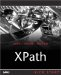Creating Quantified Expressions

Creating Quantified Expressions

Quantified expressions use the some and every keywords to perform checks on the items in a sequence. You can use the some keyword to ensure that at least one of the items in the sequence satisfies a particular criterion, and the every keyword to make sure that every item in the sequence satisfies some criterion.

Using the some Expression

Here's how you use the some quantified expression:

some  in-claus(es)  statisfies  test-expression

A quantified expression like this starts with the some keyword, followed by one or more in-clauses , followed by the keyword satisfies , followed by a test-expression . What this expression does is to let you test if at least one item in the in-clause(s) satisfies the test expression.

Let's take a look at a few examples to make this clear. This expression is true if at least one of the <planet> elements in the document has a < name > child element:

some \$planet in //planet satisfies \$planet/name

Here's an example that tests if at least one <planet> element has a language attribute:

some \$planet in //planet satisfies \$planet/@language

In this way, you can use quantified expressions to test for the existence of items like elements and attributes.

You can also test logical conditions, as here, where we're making sure that at least one planet has a mass greater than 1.5:

some \$planet in //planet satisfies (\$planet/mass > 1.5)

Here's another example:

some \$planet in //planet satisfies (\$planet/name = "Mars")

You can also use multiple in-clauses in a some expression. Here's an example, where we're testing the product of numbers :

some \$operand1 in (4, 5, 6), \$operand2 in (7, 8, 9)      satisfies \$operand1 * \$operand2 = 40

TERMINATING A some EXPRESSION

Although this is implementation-specific, the software may terminate a some expression as soon as it is able to satisfy the test expression, without evaluating all possible values.

In this case, all possible combinations of the items in the in-clauses are testednine combinations in all. That is, 4 * 7 is tested , then 4 * 8, 4 * 9, then 5 * 7, 5 * 8, and so on.

Using the every Keyword

Here's how you use the every quantified expression:

every  in-claus(es)  statifies  test-expression

This quantified expression starts with the every keyword, followed by one or more in-clauses , followed by the keyword satisfies , followed by a test-expression . This expression lets you test if every item in the in-clause(s) satisfies the test expression.

Here are a few examples. This expression is true if every one of the <planet> elements in a document has a <name> child element:

every \$planet in //planet satisfies \$planet/name

As with the some expression, you can either test for the existence of an item this way, or you can test a logical condition. This every expression tests whether planet's <day> value is greater than or equal to one:

every \$planet in //planet satisfies (\$planet/day ge 1)

And you can also use multiple in-clauses with the every expression, just as you can with some , as in this example:

every \$operand1 in (4, 5, 6), \$operand2 in (7, 8, 9)      satisfies \$operand1 * \$operand2 > 27

You can see an example where we're using every in an XSLT 2.0 stylesheet in ch08_04.xsl (Listing 8.4). In this case, we're testing three temperatures to see if they're all above 72, and if so, we'll display the message "Too hot".

Listing 8.4 An XSLT example Using the every Expression ( ch08_04.xsl )
<xsl:stylesheet version="2.0"     xmlns:xsl="http://www.w3.org/1999/XSL/Transform"     xmlns:xs="http://www.w3.org/2001/XMLSchema"> <xsl:variable name="t1" select="80" /> <xsl:variable name="t2" select="90" /> <xsl:variable name="t3" select="100" />     <xsl:template match="/">  <xsl:value-of select="if (every \$temperature in (\$t1, \$t2, \$t3)   satisfies \$temperature > 72) then 'Too hot' else 'OK'"/>  </xsl:template> </xsl:stylesheet>

Here's the result:

<?xml version="1.0" encoding="UTF-8"?> Too hotXPath Kick Start: Navigating XML with XPath 1.0 and 2.0
ISBN: 0672324113
EAN: 2147483647
Year: 2002
Pages: 131

Similar book on Amazon